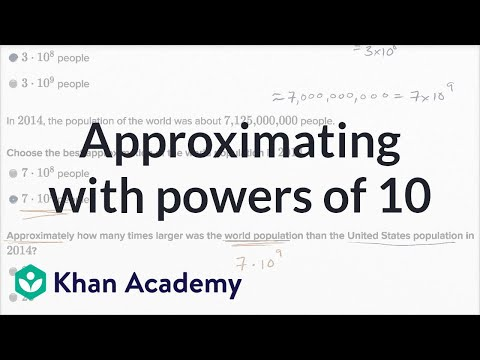Video

# Scientific notation examples (Full video)

Description: Scientific notation is a way of writing very large or very small numbers. A number is written in scientific notation when a number between 1 and 10 is multiplied by a power of 10. For example, 650,000,000 can be written in scientific notation as 6.5 × 10^8. Created by Sal Khan and CK-12 Foundation. And what I want to do in this video is just use a ck12.org Algebra I book to do some more scientific notation examples. So let's take some things that are written in scientific notation. Just as a reminder, scientific notation is useful because it allows us to write really large, or really small numbers, in ways that are easy for our brains to, one, write down, and two, understand.

### Other videos you might be interested in### Approximating with powers of 10 (Full video)